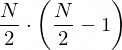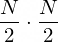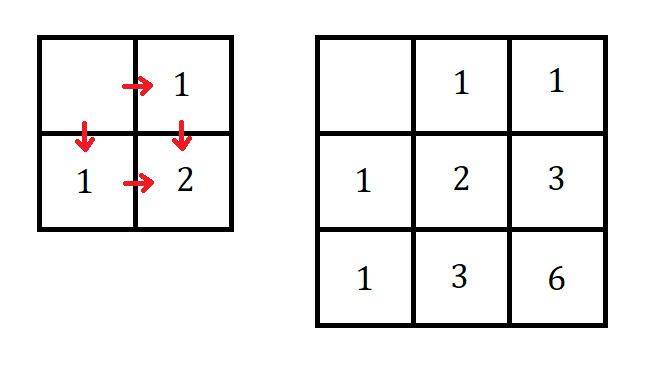# How to Solve Complicated ProblemsPhoto by Scott Graham from Unplash

There are many occasions where you can encounter a challenging problem: on an interview for a job, on a math exam, on a programming contest or even in a regular conversation with your friends. These problems are not convoluted or confusing, usually they seem surprisingly simple and, knowing the correct approach, are very easy to solve. The complicated part is finding this “correct approach” that would crack this tought nut.

Here are some of the tactics that you can use when you find yourself blocked.

# Look for Patterns

Try to find similarities between this problem and any of the previous ones you solved. Does the problem statement makes you think of a specific formula, process or equation? This advise is usually applicable in all sort of math tasks. A lot of them are designed to remind you of a specific theorem, equation or a property.

The more you know about a specific field, the easier it will be to solve this type of problems. Practice makes perfect and the big baggage of known solutions will make it easier to find another one for each new task you encounter.

## Example

Let’s look at the following example. Find the result of following product:

Try to solve it yourself first. This task has multiple solutions.

## Solution

The problem statement is supposed to remind you of difference of squares formula that looks like this.

Also let’s expand our product a little:

Now, let’s multiply our product by 1. We know for a fact that by multiplying by 1 we won’t alter the overall result. However, we will write down 1 like this:

Now we can see how multipiying by one can nicely connect with the first factor in our product. Using out formula we can multiply them together.

The result of this multiplication now connects nicely with the second factor of initial product, and so on. Following the same pattern we can say that the result of multuplying everything will be:

# Change Perspectives

In order ro solve a specific set of problems you nees to change the perspective, rephrase the problem statement or answer definition. A known example of that sort of problems are probability theory tasks. A very simple one:

The probability of a fuse fault is 0.15. There are 10 fuses in circuit. Find a probability that at least one of them will malfunction.

Calculating all posible outcomes (1 fuse malfunctioned, 2 fuses malfunctioned, 3 fuses and so on) is too long and tedious. Instead we will find a probability of circuit operating with no faults at all an subtract that from one.

In order not to get overwhelmed and find a right solution, try to organize objects or events you are countring. Categorize them into classes, filter for the ones that are important, focus on their properties and which one meet certain criterias.

Let’s look at the following problem:

I select two distinct positive whole numbers between 1 and N, where N is even, on random and add them together. What is more likely: that the resulted sum is even or odd?

Try to think how would you approach that task?

There are N positive whole numbers, so we cannot inspect every combination. However, the only quality of numbers we are interested in is it’s divisibility by 2. Just knowing that is enough since the sum of two evens will produce an even number, as well as sum of two odds. The sum of an odd and an even number will be odd.

There are as much even numbers as there are odd ones between 1 and N because N is even, so we might be tempted to say that the sum is equally likely to be either even or odd. Despite that, it’s also important we select distinct numbers. After I choose the first term, I cannot choose the same number as the second one. So, regardles whether it’s even or odd, I reduced the pool of available options of numbers by one. The total number of combinations for even sum:After selecting even or odd we reduce our options by 1.

While for the odd:After selecting an even, number of available odds stays the same.

Therefore the chances for an odd sum are higher.

## Example

N people are boarding on a plane. Unfortunately, the first passenger lost his boarding pass on his way to the cabin, so he just chooses one of the empty seats at random. The rest of passengers have their boarding passes, so they go directly to their seats. However, if they see that their seat is occupied, they choose on of the empty seats at random. When the last passenger board on the plane, what are the chances that his designted seat will be vacant?

## Solution

This task may seem challenging at first, especially given the total number of ways the boarding process can go. However, if we slightly change the definition of the task it becomes a very simple one. Imagine, that this worked just like in real life: when the person sees that their seat is occupied, they make the person stand up and look for a new one. This has no effect on which seats are occupied, but now the only person who is not sitting in their designated seat is the first passenger — they will be constatly moving on board whenever clash occures. When the last person boards the plane, the first person will be randomly seated in one of the two unclaimed seats, so there is a 50% chance that the last person will find their seat occupied.

# Split the Problem into Smaller Ones

Almost every problem you will see will be either a composition of smaller ones or just a simple ploblem stretched to big proportions. Sometimes you can quickly get the idea of how to solve the task just by reducing the dimensionality. You can try it on all previous examples, selecting small number instead of N.

Look for ways where problem can be simplified or split into parts. Focus only on significant details, reduce the numbers from statements and see if you can gain some information out of it.

## Example

There is a 10x10 grid and you are standing in the upper left corner of it. You need to get to the lower right corner, however you can only make steps down and to the right. What is the total number of paths to reach your destination?

## Solution

Let’s solve the smaller problems first. Imagine the grid to be 2x2 and 3x3. Let’s write total number of paths leading to each cell inside the cell. Then the solution will look like this:Each matrix of bigger size just copies the previous and adds a new row and a column that can be calculated from smaller matrix.

We can see that the total number of pathes can be calculated from the solutions of smaller problems. Path leading to each cell are the sum of pathes leading to the cell above and to the left. Notice that we do not keep track of how to get to the cell or what is the path, we just focus on how many ways. Knowing this we can easily calculate total number of pathes into each cell. For the matrix of size 10x10 the number in lower right corner will be 48620.

# Conclusion

Despite how frustrating complicated problems may be, the joy of solving them worth the hassle. And the more you solve them the easier they become.

There are many ways to climb a mountain and there are many solutions to a complicated task. If you can’t get to the answer, retreat, take a breath, and try to approach it from a new direction. Sooner or later the correct solution will be found.

# The final one

Try to solve the following problem:

There is an empty NxN matrix, where N is even. You and me are playing in the following game: I write any number in any empty cell, then you get to write any number in any empty cell. We do it in turns until matrix is filled. After we calculate the determinant and if it equals to zero, you win, otherwise you lose. What is your best strategy to win the game?

--

--